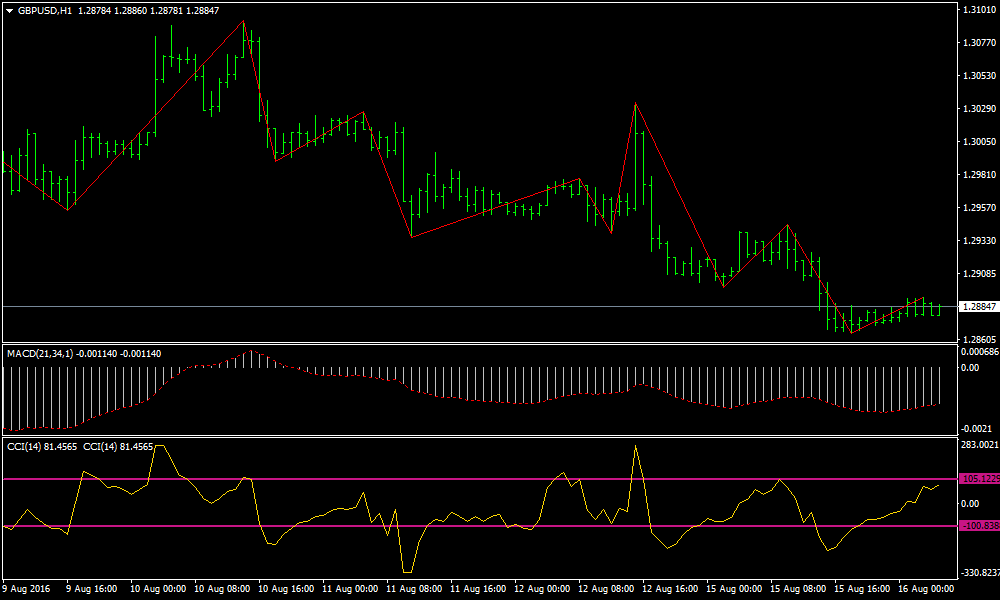## [[ Trend Following Strategies ]] MACD and CCI Forex Trend Following StrategyMACD and CCI are one of the many helpful indicators to help a trader identify signal. These are two of the basic. This system uses both indicator with the combination of zigzag indicator.

## These are the setups of the indicators:

• Zig Zag;
• MACD 21, 89, 1; set on close
• CCI 34 set on close
• CCI 46 Set on close but superimposed at the same window as CCI 34.

### Rules for Long:

• The Zig Zag must formed a low swing point.
• The CCI 34 and CCI 46 must be crossing the zero line below.
• MACD is above zero.
• If conditions 1 and 3 are met then you could enter when CCI 34 and 46 crosses the level 100.
Take profit less than 5 pips on a 5 minute chart and 8 pips in a 15 minute timeframe EU.
You can exit the position when the swing point of the Zigzag is high.

### Rules for Short:

• The Zig Zag has formed a high swing point.
• The CCI 34 and CCI 46 must be crossing the zero line above.
• MACD is below zero
• If condition 1 and 3 are met then you can enter when CCI 34 and 46 crosses the level 100 line.
Take profit less than 5 pips in a 5 minute chart, 8 pips on a 15 minute chart on a EU pair.
Exit position when the swing point of the Zig Zag is low.